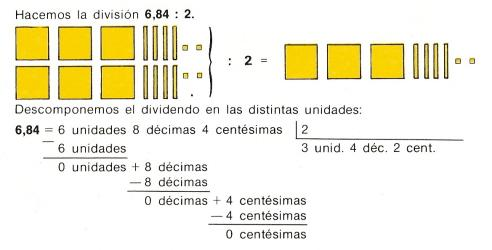6. Division (1) of decimalsIn the picture, 6.84 divided by 2 is equal to 3.42; 6.84: 2 = 3. 42

To divide a whole number by 10, we quit one zero. Example: 70: 10 = 7
To divide a whole number by 100, we quit two zeros. Example: 800: 100 = 8
To divide a whole number by 1000, we quit three zeros. Example: 5000: 1000 = 5

Solve these divisions:

 20 : 10  = 4000 : 100 = 12000 : 1000 = 1500 : 100 = 10000 : 1000 =

To divide a whole number by 10, we move the decimal point one place to the left. Example: 26:10 = 2.6
To divide a whole number by 100, we move the decimal point two places to the left. Example: 809:100 = 8, 09
To divide a whole number by 1000, we move the decimal point three places to the left. Example: 5437:1000 = 5,437

 8 : 10  = 123000 : 10 = 1027 : 100 = 6324 : 1000 = 254 : 100 =

To divide a decimal number by a natural number, we must ignore the decimal point and make the division as if the dividend and the divisor are natural numbers. Then we put the decimal point in the quotient.
Example   7,32  |_4___
33      1,83
12
0

Write these operations on a paper and solve them.

 14,52 : 3  = 10,75 : 5 = 22,512 : 6 = 5,4 : 12 = 46,17 : 9 =

Ask how formulate problemas and then solve them:

 We put in a glass 0.12 liters of water, in other glass 0. 18 and finally in another glass we put 0.17. How much water do you have in total? John's father gave 10.75 euros to his five children. How much money did each one receive? José Luis had 12.05 euros and he spent 3.25 on a pen. How much money does he have? A bag of pipes costs 0.55 euros. How much money will 7 bags cost? Peter had to walk 7.25 kilometers and he walked 3.3 kilometers in the morning. How much kilometers does he have to walk to complete his purpose? Andrew's uncle wants to distribute 14.52 euros between his three nephews. How much money will he give to each one? A notebook costs 0.35 euros. How much money will 6 notebooks cost? One bottle has 1.45 liters and another has 0.85 liters. How much liters there are in total? A teacher distributes 21.85 euros to his 19 students. How much money will he give to each one? A pencil costs 0.15 euros. How much money will 7 pencils cost?

 | Educational applications | Mathematics | In Spanish  | To print

®Arturo Ramo García.-Record of intellectual property of Teruel (Spain) No 141, of 29-IX-1999
Plaza Playa de Aro, 3, 1ş DO 44002-TERUEL